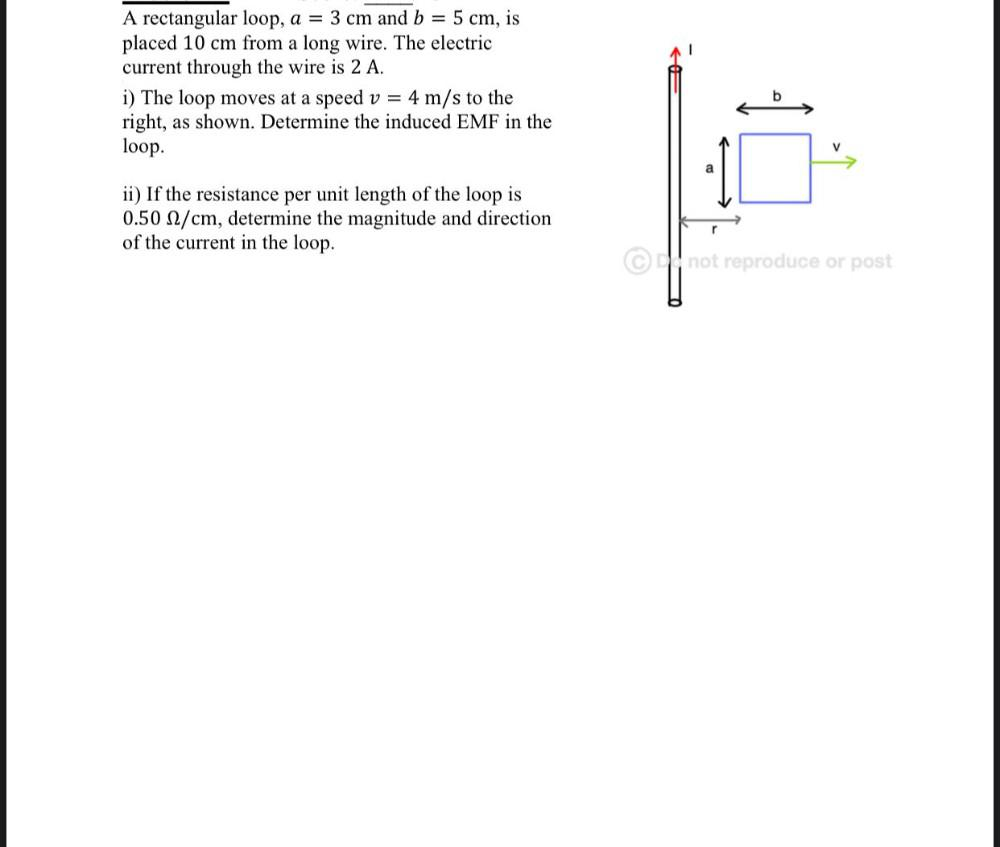Question:

# A rectangular loop, a = 3 cm and b = 5 cm, is placed 10 cm from a long wire. The electric current through the wire is 2 A. i) ThA rectangular loop, a = 3 cm and b = 5 cm, is placed 10 cm from a long wire. The electric current through the wire is 2 A. i) The loop moves at a speed v = 4 m/s to the right, as shown. Determine the induced EMF in the loop. b V ii) If the resistance per unit length of the loop is 0.50 2/cm, determine the magnitude and direction of the current in the loop. r @not reproduce or post